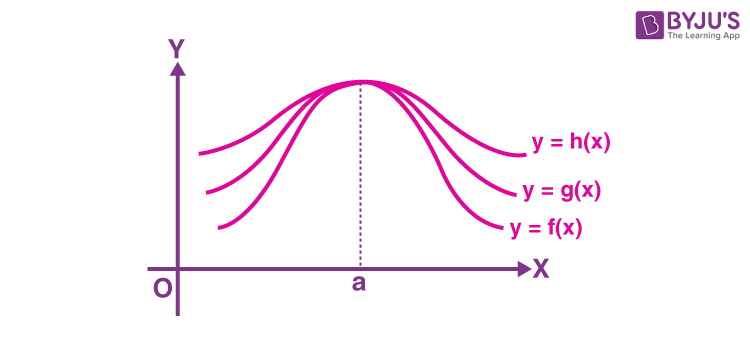# Sandwich Theorem

In Calculus, we might have come across several theorems and more specifically while determining the tricky limits and derivatives of functions. Though we have different theorems and formulas to find the limits of various types of functions, we always try to find the simpler way of solving them so that we get the solution quickly. Sandwich theorem is the one such type of application to solve limits problems. In this article, you will learn about the sandwich theorem, how to apply this theorem in solving different problems in calculus.

## Sandwich (Squeeze)Theorem

The Sandwich Theorem or squeeze theorem is used for calculating the limits of given trigonometric functions. This theorem is also known as the pinching theorem. We generally use the Sandwich theorem in calculus, including mathematical analysis. This theorem is probably used to establish the limit of a function by comparing two other functions whose limits are known or surely figured. Let’s have a look at the statement and proof of the Sandwich theorem.

Statement: Let f, g and h be real functions such that f(x) ≤ g(x) ≤ h(x) for all x in the common domain of definition. For some real number a, if $$\lim_{x\rightarrow a}f(x)=l=\lim_{x\rightarrow a}h(x)$$, then $$\lim_{x\rightarrow a}g(x)=l$$.### Sandwich Theorem proof

Let’s have a look at the geometric proof of the above statement using an inequality involving trigonometric functions.

Consider the inequality as,

cos x < sin x/x < 1 for 0 < |x| < π/2….(1)

We know that,

sin (– x) = – sin x

cos( – x) = cos x

Hence, it is sufficient to prove the above inequality for 0 < x < π/2.

For this we need to draw a unit circle.

Let O be the centre of the unit circle such that the ∠AOC is x radians and 0 < x < π/2.

Also, the line segments BA and CD are perpendiculars to OA. Finally, join AC as shown in the figure below:From the figure,

Area of ΔOAC < Area of sector OAC < Area of Δ OAB

$$\Rightarrow \frac{1}{2}\times OA\times CD <\frac{x}{2\pi}\times \pi (OA)^{2}<\frac{1}{2}\times OA\times AB$$

Here, OA is common so by cancelling the common terms, we get;

CD < x . OA < AB.

In triangle OCD,

sin x = CD/OC = CD/OA (since OC = OA as radii of same circle)

⇒ CD = OA sin x

Also,

tan x = AB/OA

⇒ AB = OA tan x

Thus,

OA sin x < OA . x < OA tan x

We know that length is always positive. So we can simplify the above inequality as:

sin x < x < tan x …..(2)

From the given, 0 < x < π/2

In this interval, sin x is positive (in the first quadrant all trigonometric functions are positive).

Dividing equ (2) by sin x,

(sin x/sin x) < (x/sin x) < (tan x/sin x)

1 < (x/sin x) < (1/cos x) {since tan x = sin x/cos x}

Taking reciprocals throughout, we get;

cos x < (sin x/x) < 1

Hence proved.

### Sandwich Theorem Limits

Using the above proof, we can also prove the following limits. The two important Sandwich theorems for limits are given below.

1. $$\lim_{x\rightarrow 0}\frac{sin x}{x}=1$$
2. $$\lim_{x\rightarrow 0}\frac{1-cos x}{x}=0$$

Based on these limits, we can prove different identities involving limits and trigonometry functions.

### Sandwich Theorem Example

Question:

Evaluate $$\lim_{x\rightarrow 0}\frac{sin\ 4x}{sin\ 2x}$$ using the Sandwich theorem.

Solution:

$$\lim_{x\rightarrow 0}\frac{sin\ 4x}{sin\ 2x}$$

Multiplying and dividing by 4x,

$$=\lim_{x\rightarrow 0}\frac{sin\ 4x}{4x}\times \frac{2x}{sin\ 2x}\times 2$$

Take the constant out of the limit,

$$=2\times \lim_{x\rightarrow 0}\frac{(\frac{sin\ 4x}{4x})}{(\frac{sin\ 2x}{2x})}$$

We can write the numerator part as:

$$\lim_{4x\rightarrow 0}\frac{sin\ 4x}{4x} = 1$$ {since x → 0, 4x → 0 and 2x → 0 are the same}

Similarly, the denominator part will be:

$$\lim_{2x\rightarrow 0}\frac{sin\ 2x}{2x} = 1$$

Thus,

$$2\times \lim_{x\rightarrow 0}\frac{(\frac{sin\ 4x}{4x})}{(\frac{sin\ 2x}{2x})}= 2\times \frac{1}{1}=1$$

Therefore, $$\lim_{x\rightarrow 0}\frac{sin\ 4x}{sin\ 2x}=1$$

For more interesting theorems in calculus, visit www.byjus.com and download BYJU’S – The Learning App for interactive videos for maths concepts.